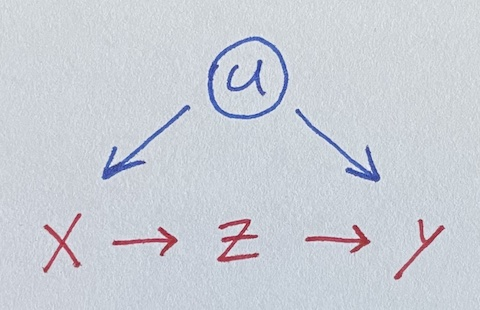# Simple Front Door Regression

Saturday May 1, 2021

If you have a mediator, you can estimate effects despite confounding, which is neat. The idea is like flip of instrumental variables; here the constructed non-confounded variable is what’s not explained by the confounded one. A two-stage regression illustrates the idea.

We seek to estimate the impact of x on y, where they’re both influenced by u.Unfortunately, u is unobserved, so we can’t control for it. But lo, there is z, a perfect mediator between x and y. Let’s simulate data where all the true coefficients are one.

``````u = rnorm(100)
x = u + rnorm(100)
z = x + rnorm(100)
y = u + z + rnorm(100)``````

Regressing naively, we get an incorrect estimate for the effect of x on y.

``````summary(lm(y ~ x))
##              Estimate Std. Error t value Pr(>|t|)
##  x            1.54648    0.09848  15.704   <2e-16 ***``````

Luckily, z is in there, and it isn’t confounded with u.

But how do we use it? Throwing it in the regression doesn’t help.

``````summary(lm(y ~ x + z))
##              Estimate Std. Error t value Pr(>|t|)
##  x            0.46175    0.15517   2.976  0.00369 **
##  z            1.02436    0.12740   8.041  2.2e-12 ***``````

A fancier model-fitting approach is needed. Here’s an illustrative two-stage regression, ignoring standard error concerns.

Stage one: Do a regression using x to predict z. Note the coefficient on x. Then get the residuals for z from that model, which I’ll call z_not_from_x. This uses the non-confounding of z with u to make a “version of” (maybe a component of) z that is independent of u (because it’s independent of x): the variation of z not due to x (or u).

Stage two: Do a regression using z_not_from_x to predict y.

Multiplying the coefficients from the two models gives the effect of x on y.

``````summary(lm(z ~ x))
##              Estimate Std. Error t value Pr(>|t|)
##  x           1.058940   0.060797  17.418   <2e-16 ***
z_not_from_x = residuals(lm(z ~ x))
summary(lm(y ~ z_not_from_x))
##               Estimate Std. Error t value Pr(>|t|)
##  z_not_from_x   1.0244     0.2889   3.546 0.000601 ***
1.058940 * 1.0244  # Estimate for x on y
##  1.084778``````

We’ve recovered a fair estimate of the true parameter for x, despite not using the unobserved confound u!

Here’s the same thing as above but with the `lavaan` package for SEM, following Thoemmes.

``````model = "z ~ x_on_z * x
y ~ z_on_y * z
x ~~ y  # Allow x and y to still covary
x_on_y := x_on_z * z_on_y"
summary(sem(model, data.frame(x=x, z=z, y=y)))
##                   Estimate  Std.Err  z-value  P(>|z|)
##    x_on_z            1.059    0.060   17.594    0.000
##    z_on_y            1.024    0.125    8.164    0.000
##    x_on_y            1.085    0.146    7.406    0.000``````

So there’s standard errors for you!

### Other cases

This example goes well with a collection of four simpler ("back door") regression situations, What should be in your regression? It goes especially well with its cousin, A simple Instrumental Variable.

### More complicated cases

The dagitty tools seems to be a great way to analyze a given situation (expressed as a DAG) and figure out what you can do with it.

Maybe regression discontinuity is another kind of case?